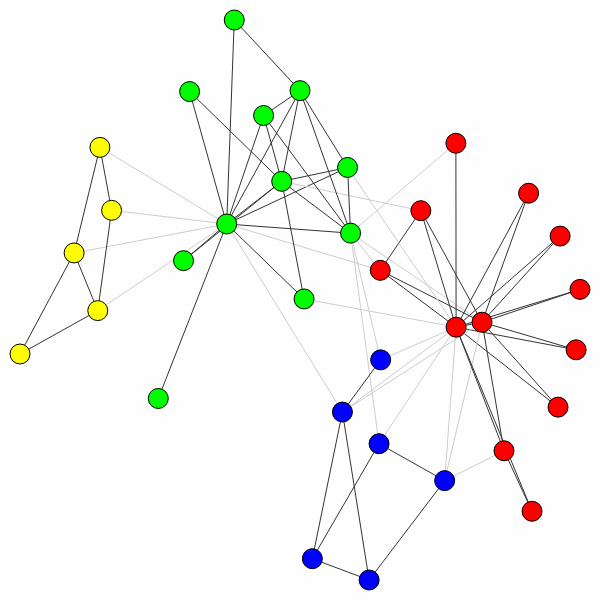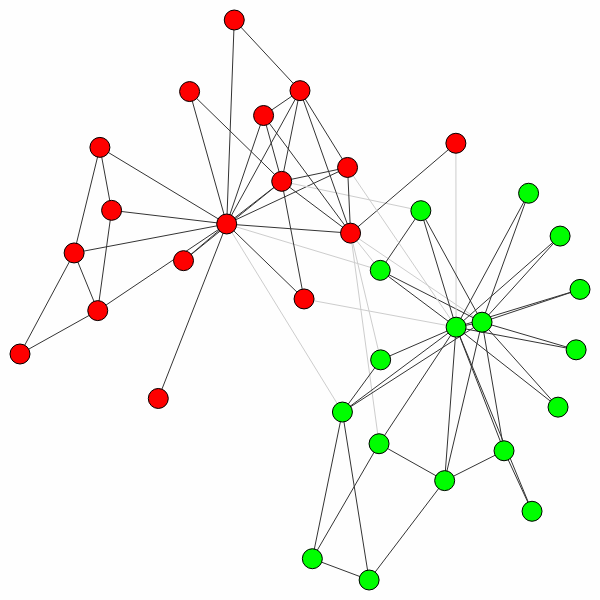Introduction¶

This package facilitates community detection of networks and builds on the package igraph, referred to as ig throughout this documentation. Although the options in the package are extensive, most people are presumably simply interested in detecting communities with a robust method that works well. This introduction explains how to do that.

For those without patience (and some prior experience), if you simply want to detect communities given a graph G using modularity, you simply use

>>> partition = louvain.find_partition(G, louvain.ModularityVertexPartition);

That’s it.

Why then should you use this package rather than the Louvain algorithm community_multilevel() built into igraph? If you want to use modularity, and you work with a simple undirected, unweighted graph, then indeed you may use the built-in method. For anything else, the functionality is not built-in and this package is for you.

For those less familiar with igraph, let us work out an example more fully. First, we need to import the relevant packages:

>>> import igraph as ig
>>> import louvain

Let us then look at one of the most famous examples of network science: the Zachary karate club (it even has a prize named after it):

>>> G = ig.Graph.Famous('Zachary')

Now detecting communities with modularity is straightforward, as demonstrated earlier:

>>> partition = louvain.find_partition(G, louvain.ModularityVertexPartition)

You can simply plot the results as follows:

>>> ig.plot(partition)In this case, the algorithm actually finds the optimal partition (for small graphs like these you can check this using community_optimal_modularity() in the igraph package), but this is generally not the case (although the algorithm should do well). Although this is the optimal partition, it does not correspond to the split in two factions that was observed for this particular network. We can uncover that split in two using a different method: CPMVertexPartition:

>>> partition = louvain.find_partition(G, louvain.CPMVertexPartition,
...                                    resolution_parameter = 0.05);
>>> ig.plot(partition)Note that any additional **kwargs passed to find_partition() is passed on to the constructor of the given partition_type. In this case, we can pass the resolution_parameter, but we could also pass weights or node_sizes.

This is the real benefit of using this package: it provides implementations for six different methods (see Reference), and works also on directed and weighted graphs. Finally, it also allows to work with more complex multiplex graphs (see Multiplex).